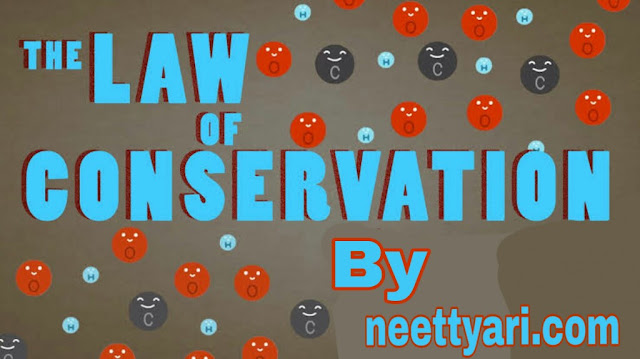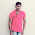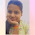In any physical phenomena taking in nature, several quantities change with time, while some special physical quantities remain constant with time. The later quantities are 'conserved quantities' of nature, and are governed by certain laws of conservation.Conservation laws in Physics

Some of the general laws of conservation on which we are going to discuss in this post are as follows :-

## Laws of Conservations of nature in Physics

### 1) Conservation of Mechanical Energy

For motion under an external conservative force (A conservative force is a force with the property that the total work done in moving a particle between two points is independent of the taken path), the mechanical energy (Kinetic energy + Potential energy) of a body is a constant.

### 2) Conservation of Total Energy of an Isolated System

The mechanical energy is conserved if the forces involved are conservative. If some of the forces doing work non-conservative (such as air friction), part of the mechanical energy transformed into other forms.

### 3) Conservation of Mass Plus Energy

Before Einstein, the law of conservation of mass was considered as a basic law in nature because matter was thought to be indestructible. But, Einstein, by his theory of relativity proved that energy conservation and mass conservation are not two independent laws ; they are unified in a single law. According to it, the total (mass + energy) of the universe is conserved.

∆E = (∆m) c^2

### 4) Conservation of Momentum

Energy is a scaler quantity. All conserved quantities are, however, not necessarily scalers. The total linear momentum and the total angular momentum (both vector) of an isolated system are also conserved quantities. These laws are derived from Newtown laws of motion in mechanics. These are the basic conservation laws of nature in all domains.

### 5) Conservation of Charge

Conservation of electric charge of an isolated system is a fundamental law of nature. The total amount of charge in an isolated system remains constant. It means charge can neither be created nor destroyed.

### 6) Conservation of Mass Number

In all nuclear reactions, the number of nucleons remains conserved. There is rearrangement of protons and neutrons in different nuclear reactions, but the total number of neutrons and protons is separately equal for the reactants and the products.

1.1.